Скачать презентацию CDAE 272 International Economic Development Spring 2008

22153e5cc630815446e01582d450fd62.ppt

• Количество слайдов: 19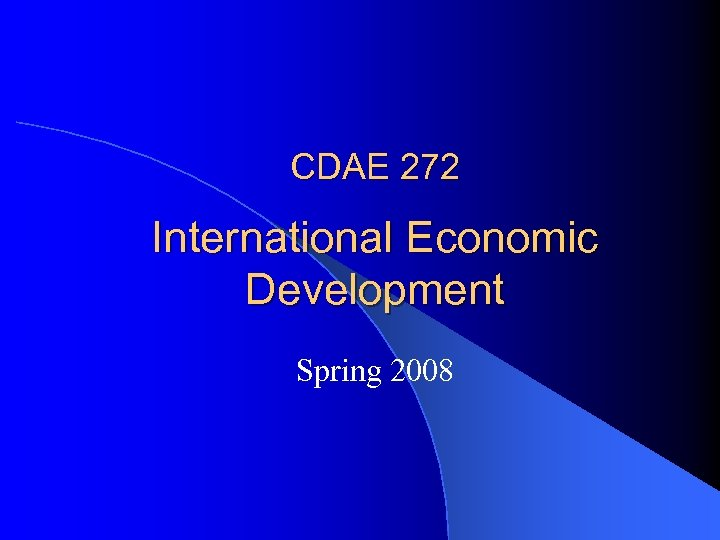CDAE 272 International Economic Development Spring 2008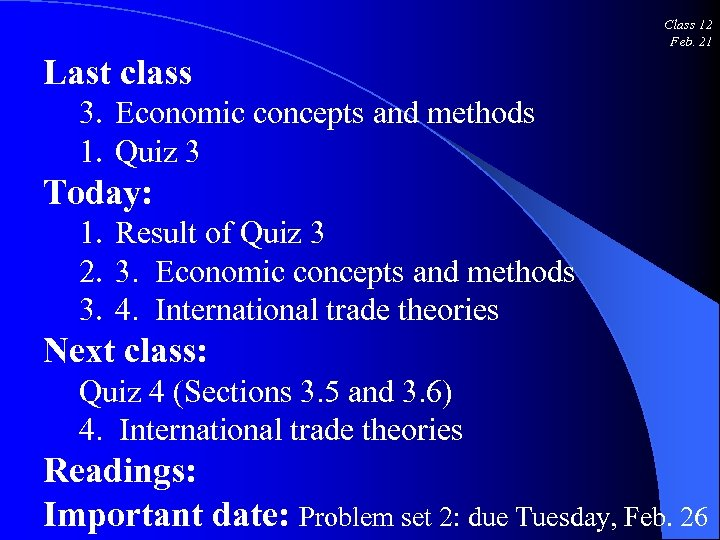Class 12 Feb. 21 Last class 3. Economic concepts and methods 1. Quiz 3 Today: 1. Result of Quiz 3 2. 3. Economic concepts and methods 3. 4. International trade theories Next class: Quiz 4 (Sections 3. 5 and 3. 6) 4. International trade theories Readings: Important date: Problem set 2: due Tuesday, Feb. 26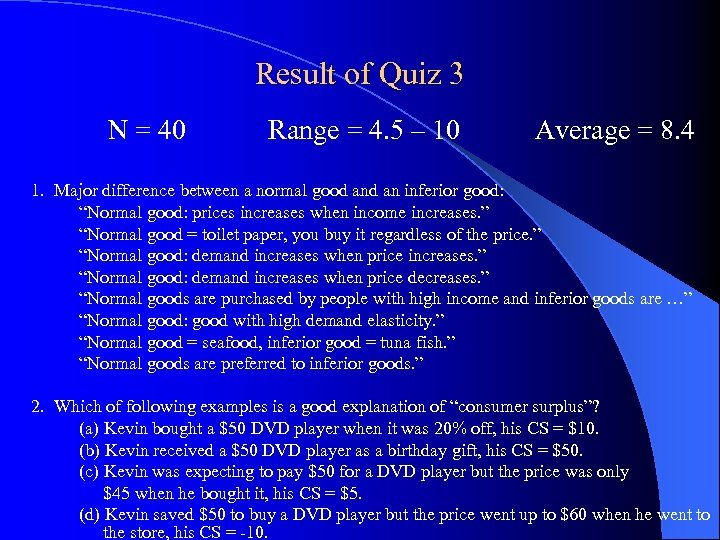Result of Quiz 3 N = 40 Range = 4. 5 – 10 Average = 8. 4 1. Major difference between a normal good an inferior good: “Normal good: prices increases when income increases. ” “Normal good = toilet paper, you buy it regardless of the price. ” “Normal good: demand increases when price increases. ” “Normal good: demand increases when price decreases. ” “Normal goods are purchased by people with high income and inferior goods are …” “Normal good: good with high demand elasticity. ” “Normal good = seafood, inferior good = tuna fish. ” “Normal goods are preferred to inferior goods. ” 2. Which of following examples is a good explanation of “consumer surplus”? (a) Kevin bought a \$50 DVD player when it was 20% off, his CS = \$10. (b) Kevin received a \$50 DVD player as a birthday gift, his CS = \$50. (c) Kevin was expecting to pay \$50 for a DVD player but the price was only \$45 when he bought it, his CS = \$5. (d) Kevin saved \$50 to buy a DVD player but the price went up to \$60 when he went to the store, his CS = -10.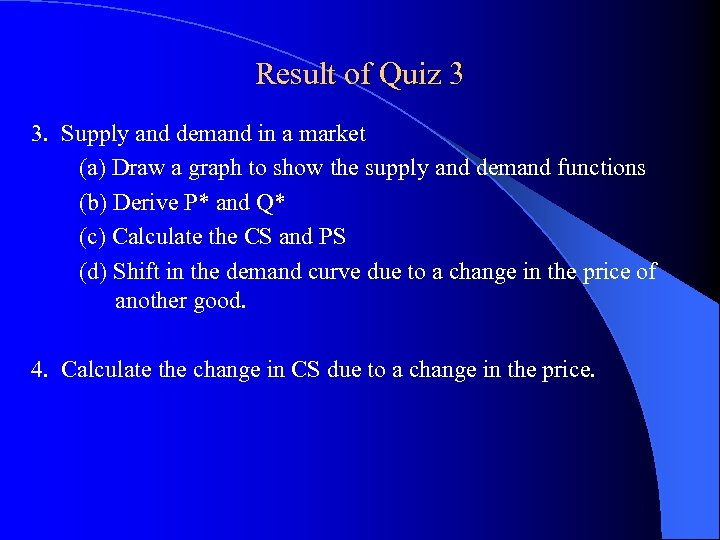Result of Quiz 3 3. Supply and demand in a market (a) Draw a graph to show the supply and demand functions (b) Derive P* and Q* (c) Calculate the CS and PS (d) Shift in the demand curve due to a change in the price of another good. 4. Calculate the change in CS due to a change in the price.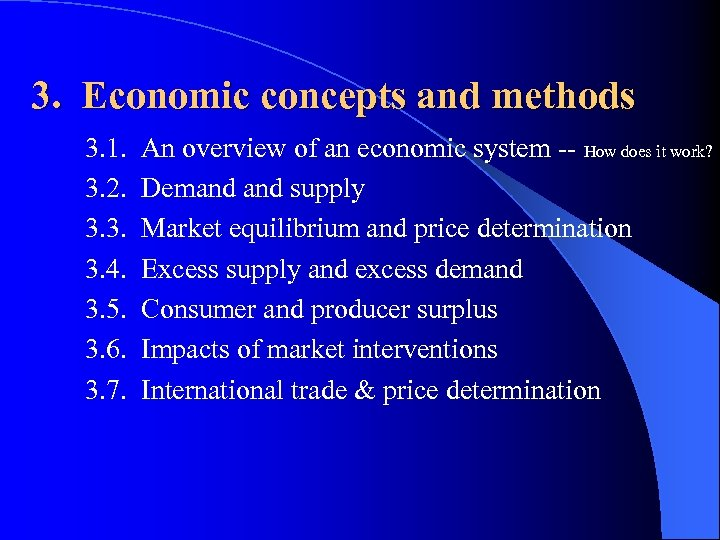3. Economic concepts and methods 3. 1. 3. 2. 3. 3. 3. 4. 3. 5. 3. 6. 3. 7. An overview of an economic system -- How does it work? Demand supply Market equilibrium and price determination Excess supply and excess demand Consumer and producer surplus Impacts of market interventions International trade & price determination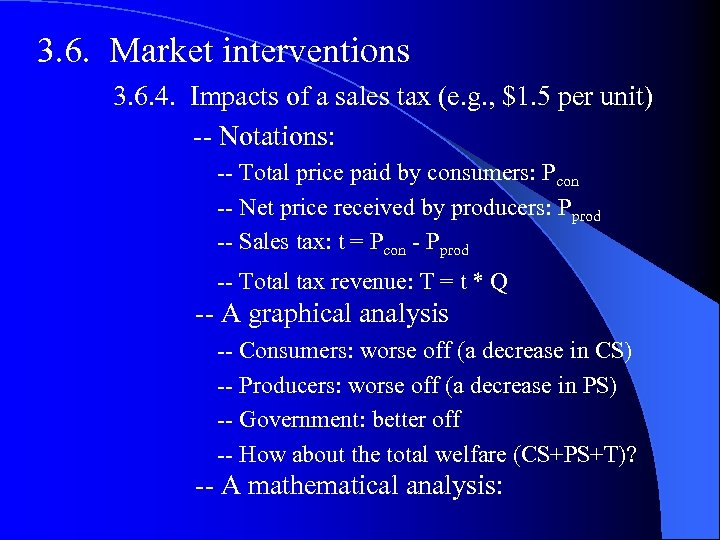3. 6. Market interventions 3. 6. 4. Impacts of a sales tax (e. g. , \$1. 5 per unit) -- Notations: -- Total price paid by consumers: Pcon -- Net price received by producers: Pprod -- Sales tax: t = Pcon - Pprod -- Total tax revenue: T = t * Q -- A graphical analysis -- Consumers: worse off (a decrease in CS) -- Producers: worse off (a decrease in PS) -- Government: better off -- How about the total welfare (CS+PS+T)? -- A mathematical analysis: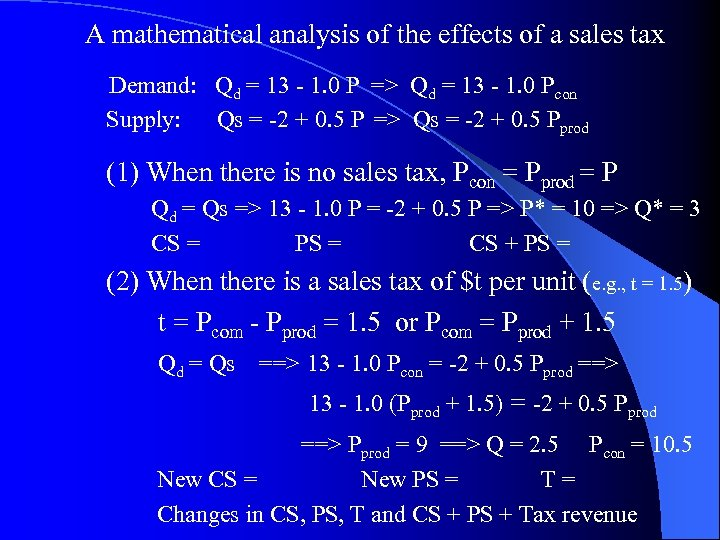A mathematical analysis of the effects of a sales tax Demand: Qd = 13 - 1. 0 P => Qd = 13 - 1. 0 Pcon Supply: Qs = -2 + 0. 5 P => Qs = -2 + 0. 5 Pprod (1) When there is no sales tax, Pcon = Pprod = P Qd = Qs => 13 - 1. 0 P = -2 + 0. 5 P => P* = 10 => Q* = 3 CS = PS = CS + PS = (2) When there is a sales tax of \$t per unit (e. g. , t = 1. 5) t = Pcom - Pprod = 1. 5 or Pcom = Pprod + 1. 5 Qd = Qs ==> 13 - 1. 0 Pcon = -2 + 0. 5 Pprod ==> 13 - 1. 0 (Pprod + 1. 5) = -2 + 0. 5 Pprod ==> Pprod = 9 ==> Q = 2. 5 Pcon = 10. 5 New CS = New PS = T= Changes in CS, PS, T and CS + PS + Tax revenue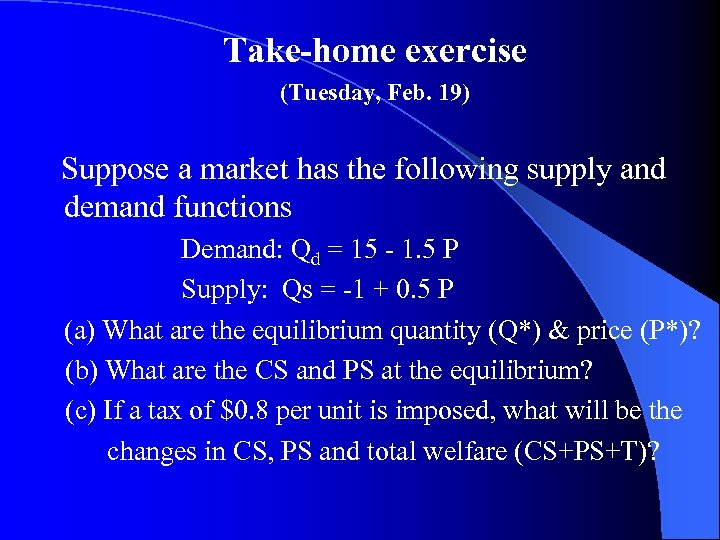Take-home exercise (Tuesday, Feb. 19) Suppose a market has the following supply and demand functions Demand: Qd = 15 - 1. 5 P Supply: Qs = -1 + 0. 5 P (a) What are the equilibrium quantity (Q*) & price (P*)? (b) What are the CS and PS at the equilibrium? (c) If a tax of \$0. 8 per unit is imposed, what will be the changes in CS, PS and total welfare (CS+PS+T)?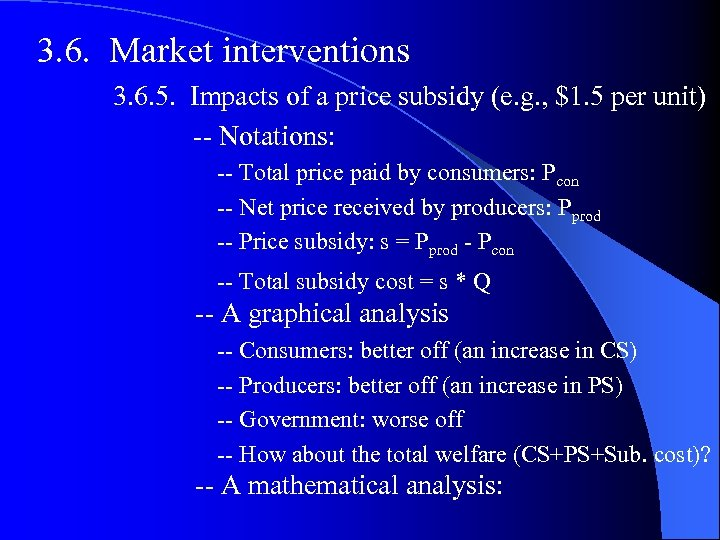3. 6. Market interventions 3. 6. 5. Impacts of a price subsidy (e. g. , \$1. 5 per unit) -- Notations: -- Total price paid by consumers: Pcon -- Net price received by producers: Pprod -- Price subsidy: s = Pprod - Pcon -- Total subsidy cost = s * Q -- A graphical analysis -- Consumers: better off (an increase in CS) -- Producers: better off (an increase in PS) -- Government: worse off -- How about the total welfare (CS+PS+Sub. cost)? -- A mathematical analysis: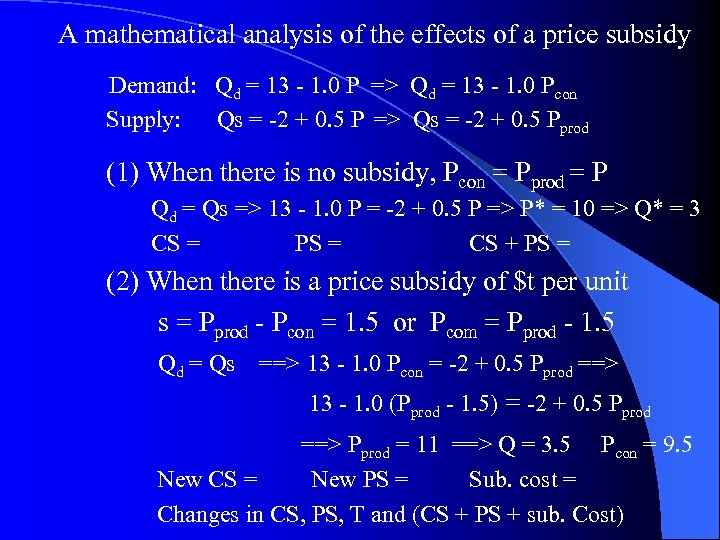A mathematical analysis of the effects of a price subsidy Demand: Qd = 13 - 1. 0 P => Qd = 13 - 1. 0 Pcon Supply: Qs = -2 + 0. 5 P => Qs = -2 + 0. 5 Pprod (1) When there is no subsidy, Pcon = Pprod = P Qd = Qs => 13 - 1. 0 P = -2 + 0. 5 P => P* = 10 => Q* = 3 CS = PS = CS + PS = (2) When there is a price subsidy of \$t per unit s = Pprod - Pcon = 1. 5 or Pcom = Pprod - 1. 5 Qd = Qs ==> 13 - 1. 0 Pcon = -2 + 0. 5 Pprod ==> 13 - 1. 0 (Pprod - 1. 5) = -2 + 0. 5 Pprod ==> Pprod = 11 ==> Q = 3. 5 Pcon = 9. 5 New CS = New PS = Sub. cost = Changes in CS, PS, T and (CS + PS + sub. Cost)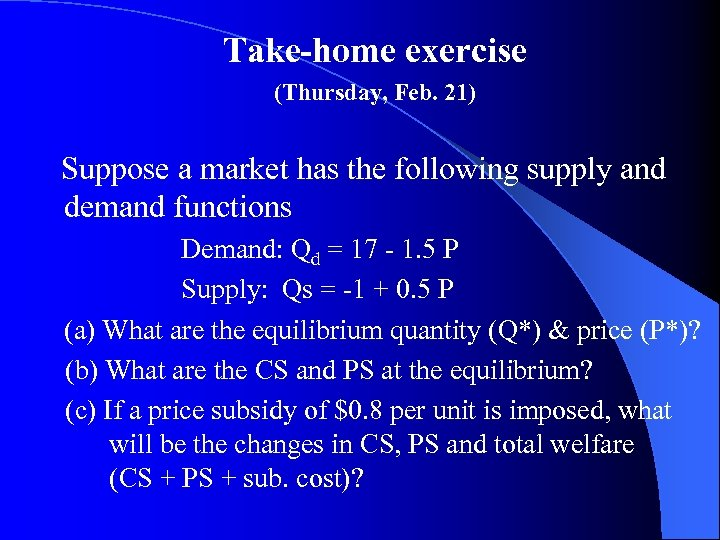Take-home exercise (Thursday, Feb. 21) Suppose a market has the following supply and demand functions Demand: Qd = 17 - 1. 5 P Supply: Qs = -1 + 0. 5 P (a) What are the equilibrium quantity (Q*) & price (P*)? (b) What are the CS and PS at the equilibrium? (c) If a price subsidy of \$0. 8 per unit is imposed, what will be the changes in CS, PS and total welfare (CS + PS + sub. cost)?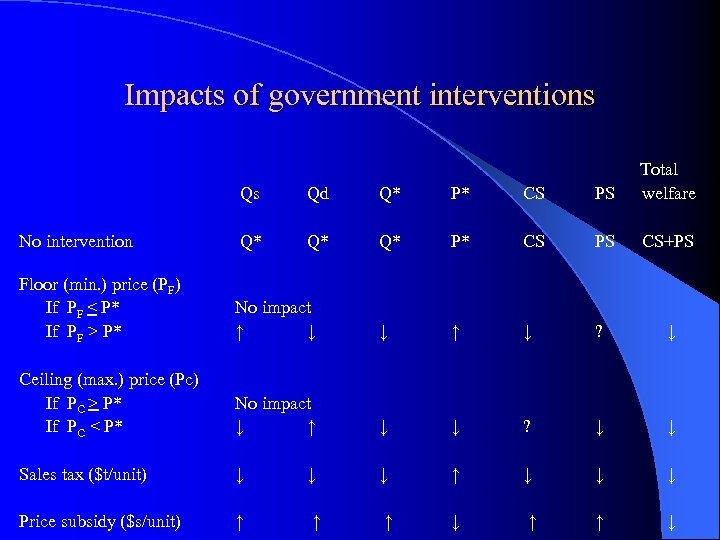Impacts of government interventions Qs Qd Q* P* CS PS Total welfare No intervention Q* Q* Q* P* CS PS CS+PS Floor (min. ) price (PF) If PF < P* If PF > P* No impact ↑ ↓ ↓ ↑ ↓ ? ↓ Ceiling (max. ) price (Pc) If PC > P* If PC < P* No impact ↓ ↑ ↓ ↓ ? ↓ ↓ Sales tax (\$t/unit) ↓ ↓ ↓ ↑ ↓ ↓ ↓ Price subsidy (\$s/unit) ↑ ↑ ↑ ↓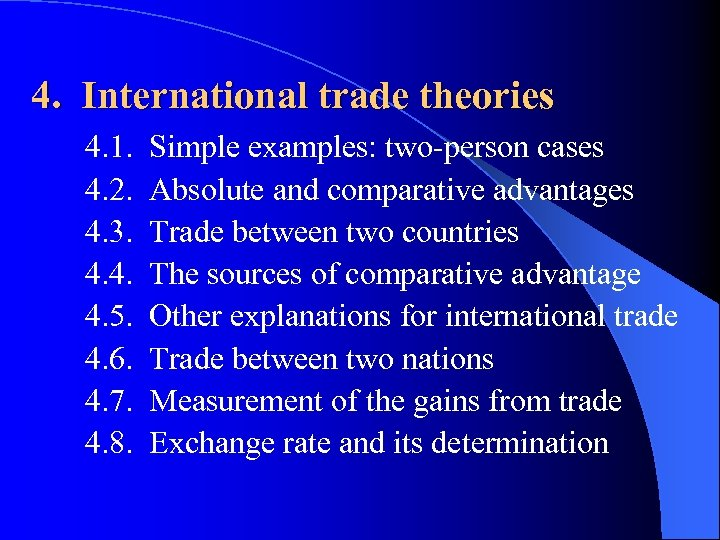4. International trade theories 4. 1. 4. 2. 4. 3. 4. 4. 4. 5. 4. 6. 4. 7. 4. 8. Simple examples: two-person cases Absolute and comparative advantages Trade between two countries The sources of comparative advantage Other explanations for international trade Trade between two nations Measurement of the gains from trade Exchange rate and its determination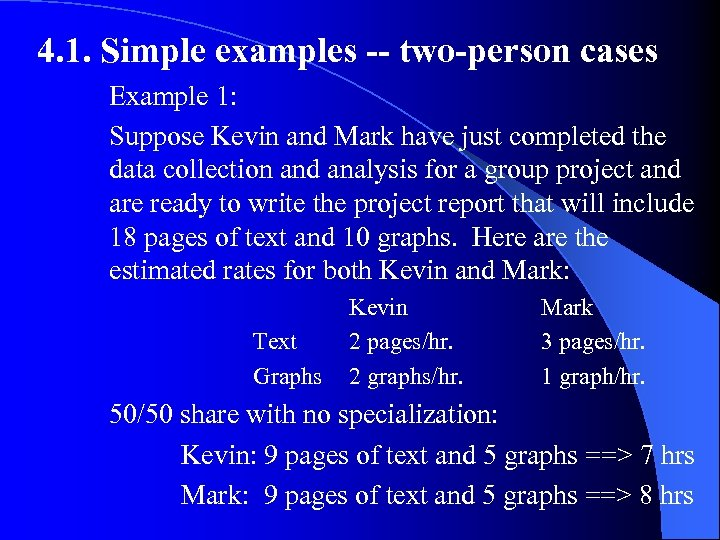4. 1. Simple examples -- two-person cases Example 1: Suppose Kevin and Mark have just completed the data collection and analysis for a group project and are ready to write the project report that will include 18 pages of text and 10 graphs. Here are the estimated rates for both Kevin and Mark: Text Graphs Kevin 2 pages/hr. 2 graphs/hr. Mark 3 pages/hr. 1 graph/hr. 50/50 share with no specialization: Kevin: 9 pages of text and 5 graphs ==> 7 hrs Mark: 9 pages of text and 5 graphs ==> 8 hrs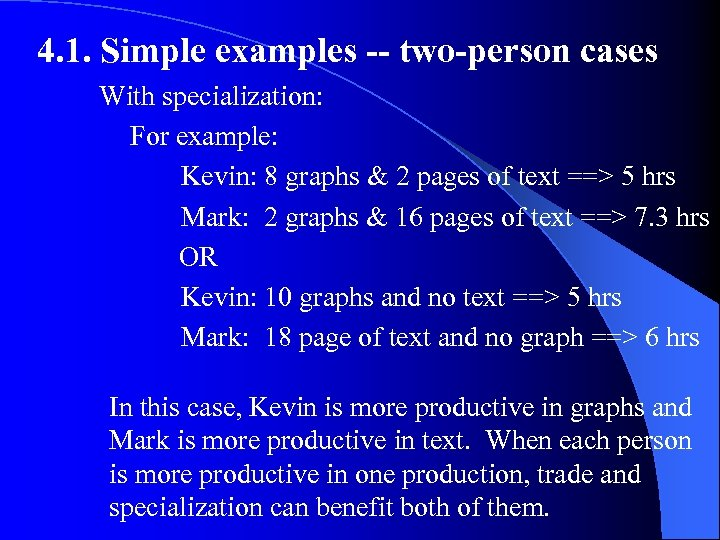4. 1. Simple examples -- two-person cases With specialization: For example: Kevin: 8 graphs & 2 pages of text ==> 5 hrs Mark: 2 graphs & 16 pages of text ==> 7. 3 hrs OR Kevin: 10 graphs and no text ==> 5 hrs Mark: 18 page of text and no graph ==> 6 hrs In this case, Kevin is more productive in graphs and Mark is more productive in text. When each person is more productive in one production, trade and specialization can benefit both of them.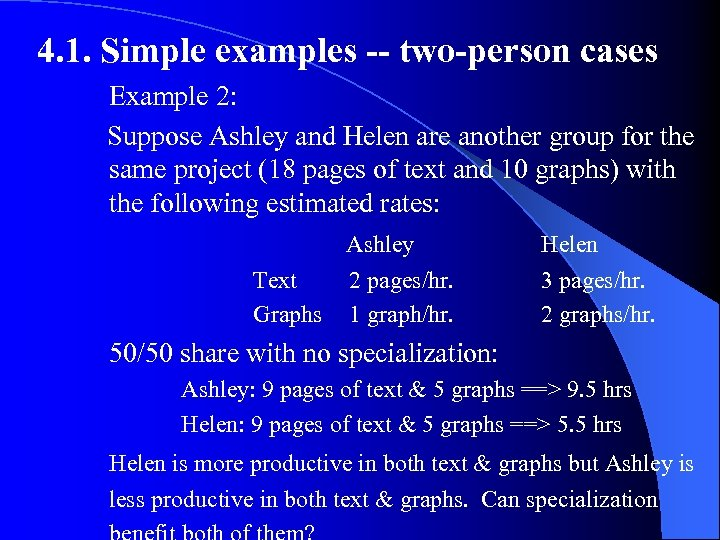4. 1. Simple examples -- two-person cases Example 2: Suppose Ashley and Helen are another group for the same project (18 pages of text and 10 graphs) with the following estimated rates: Ashley Text 2 pages/hr. Graphs 1 graph/hr. Helen 3 pages/hr. 2 graphs/hr. 50/50 share with no specialization: Ashley: 9 pages of text & 5 graphs ==> 9. 5 hrs Helen: 9 pages of text & 5 graphs ==> 5. 5 hrs Helen is more productive in both text & graphs but Ashley is less productive in both text & graphs. Can specialization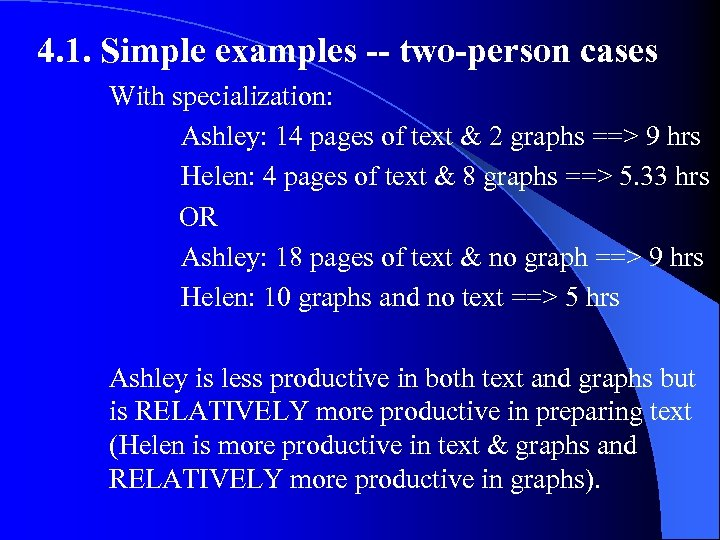4. 1. Simple examples -- two-person cases With specialization: Ashley: 14 pages of text & 2 graphs ==> 9 hrs Helen: 4 pages of text & 8 graphs ==> 5. 33 hrs OR Ashley: 18 pages of text & no graph ==> 9 hrs Helen: 10 graphs and no text ==> 5 hrs Ashley is less productive in both text and graphs but is RELATIVELY more productive in preparing text (Helen is more productive in text & graphs and RELATIVELY more productive in graphs).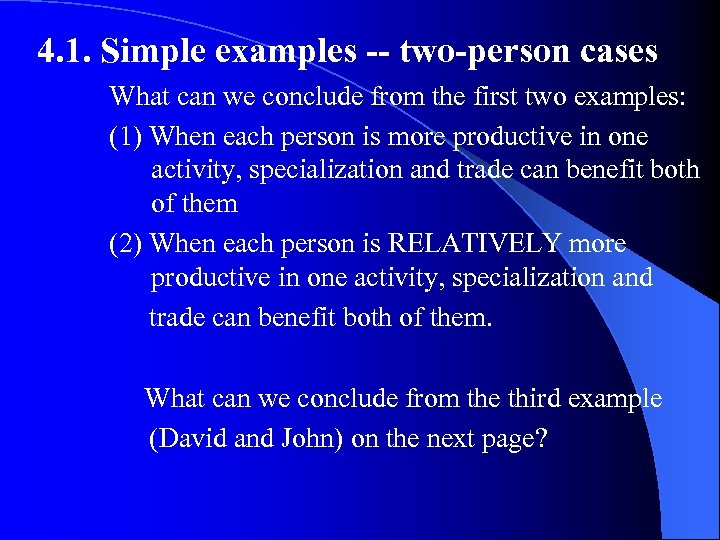4. 1. Simple examples -- two-person cases What can we conclude from the first two examples: (1) When each person is more productive in one activity, specialization and trade can benefit both of them (2) When each person is RELATIVELY more productive in one activity, specialization and trade can benefit both of them. What can we conclude from the third example (David and John) on the next page?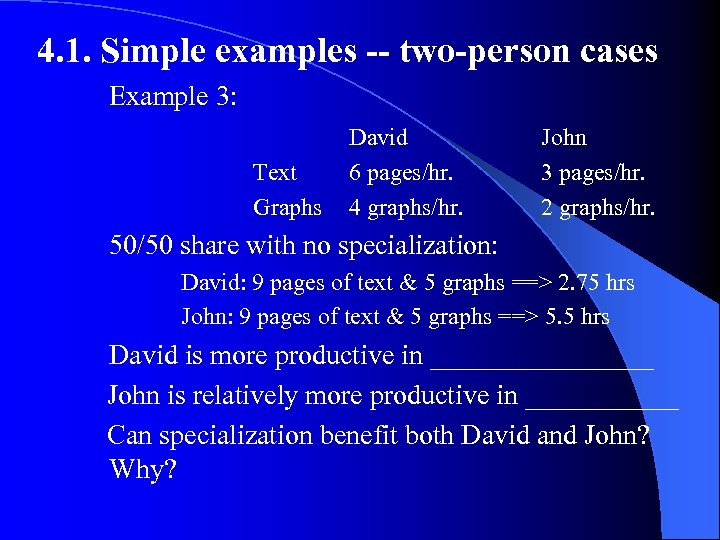4. 1. Simple examples -- two-person cases Example 3: Text Graphs David 6 pages/hr. 4 graphs/hr. John 3 pages/hr. 2 graphs/hr. 50/50 share with no specialization: David: 9 pages of text & 5 graphs ==> 2. 75 hrs John: 9 pages of text & 5 graphs ==> 5. 5 hrs David is more productive in ________ John is relatively more productive in ______ Can specialization benefit both David and John? Why?